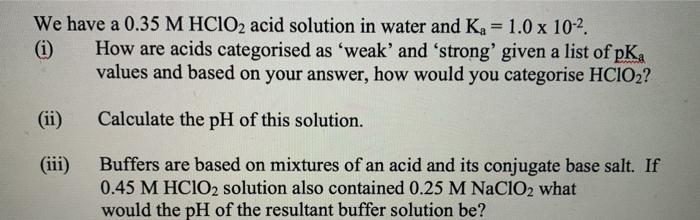### Create an Account

Home / Questions / We have a 0.35 M HClO2 acid solution in water and K = 1.0 x 10-2. (1) How are acids catego...

# We have a 0.35 M HClO2 acid solution in water and K = 1.0 x 10-2. (1) How are acids categorised as 'weak' and 'strong' given a list of pKa values and based on your answer, how would you categorise

We have a 0.35 M HClO2 acid solution in water and K = 1.0 x 10-2. (1) How are acids categorised as 'weak' and 'strong' given a list of pKa values and based on your answer, how would you categorise HCIO2? Calculate the pH of this solution. (iii) Buffers are based on mixtures of an acid and its conjugate base salt. If 0.45 M HClO2 solution also contained 0.25 M NaClO2 what would the pH of the resultant buffer solution be?

\May 17 2021 View more View LessSubscribe To Get Solution